# Partial differential equation

In mathematics, a partial differential equation (PDE) is an equation which imposes relations between the various partial derivatives of a multivariable function. The function is often thought of as an "unknown" to be solved for, similarly to how x is thought of as an unknown number to be solved for in an algebraic equation like x2 − 3x + 2 = 0. However, it is usually impossible to write down explicit formulas for solutions of partial differential equations. There is, correspondingly, a vast amount of modern mathematical and scientific research on methods to numerically approximate solutions of certain partial differential equations using computers. Partial differential equations also occupy a large sector of pure mathematical research, in which the usual questions are, broadly speaking, on the identification of general qualitative features of solutions of various partial differential equations, such as existence, uniqueness, regularity, and stability. Among the many open questions are the existence and smoothness of solutions to the Navier–Stokes equations, named as one of the Millennium Prize Problems in 2000. Partial differential equations are ubiquitous in mathematically oriented scientific fields, such as physics and engineering. For instance, they are foundational in the modern scientific understanding of sound, heat, diffusion, electrostatics, electrodynamics, thermodynamics, fluid dynamics, elasticity, general relativity, and quantum mechanics (Schrödinger equation, Pauli equation, etc). They also arise from many purely mathematical considerations, such as differential geometry and the calculus of variations; among other notable applications, they are the fundamental tool in the proof of the Poincaré conjecture from geometric topology. Partly due to this variety of sources, there is a wide spectrum of different types of partial differential equations, and methods have been developed for dealing with many of the individual equations which arise. As such, it is usually acknowledged that there is no "general theory" of partial differential equations, with specialist knowledge being somewhat divided between several essentially distinct subfields. Ordinary differential equations form a subclass of partial differential equations, corresponding to functions of a single variable. Stochastic partial differential equations and nonlocal equations are, as of 2020, particularly widely studied extensions of the "PDE" notion. More classical topics, on which there is still much active research, include elliptic and parabolic partial differential equations, fluid mechanics, Boltzmann equations, and dispersive partial differential equations. (Wikipedia).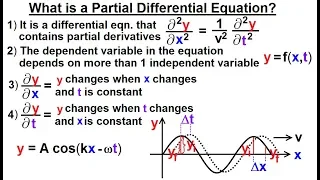Math: Partial Differential Eqn. - Ch.1: Introduction (1 of 42) What is a Partial Differential Eqn?

Visit http://ilectureonline.com for more math and science lectures! In this video I will explain what is a partial differential equation. PDE is a differential equation that contains partial derivatives, and the dependent variable in the equation depends on more than 1 independent variab

From playlist PARTIAL DIFFERENTIAL EQNS CH1 INTRODUCTIONWhat are differential equations?

► My Differential Equations course: https://www.kristakingmath.com/differential-equations-course Differential equations are usually classified into two general categories: partial differential equations, which are also called partial derivatives, and ordinary differential equations. Part

From playlist Popular QuestionsIntroduction to Differential Equations

Please Subscribe here, thank you!!! https://goo.gl/JQ8Nys Introduction to Differential Equations - The types of differential equations, ordinary versus partial. - How to find the order of a differential equation.

From playlist Differential Equations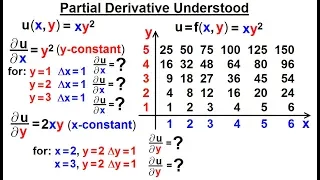Math: Partial Differential Eqn. - Ch.1: Introduction (6 of 42) Partial Derivative Understood

Visit http://ilectureonline.com for more math and science lectures! In this video I will further explain what is partial differential equation by using a graphical example to explain what is a partial derivative of the equation u=f(x,y)=xy^2. Next video in this series can be seen at: ht

From playlist PARTIAL DIFFERENTIAL EQNS CH1 INTRODUCTION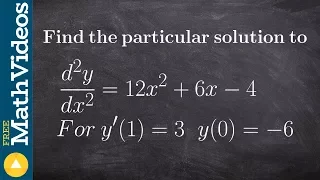Find the particular solution given the conditions and second derivative

Learn how to solve the particular solution of differential equations. A differential equation is an equation that relates a function with its derivatives. The solution to a differential equation involves two parts: the general solution and the particular solution. The general solution give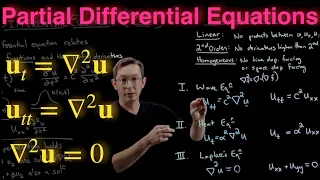Partial Differential Equations Overview

Partial differential equations are the mathematical language we use to describe physical phenomena that vary in space and time. Examples include gravitation, electromagnetism, and fluid dynamics. @eigensteve on Twitter eigensteve.com databookuw.com %%% CHAPTERS %%% 0:00 Overview of Pa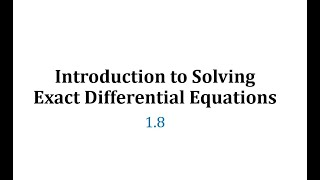(1.8) Introduction to Solving Exact Differential Equations

This video introduces and explains how to solve an exact differential equation. https://mathispower4u.com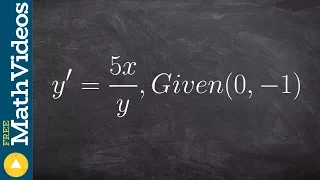How to solve a differentialble equation by separating the variables

Learn how to solve the particular solution of differential equations. A differential equation is an equation that relates a function with its derivatives. The solution to a differential equation involves two parts: the general solution and the particular solution. The general solution give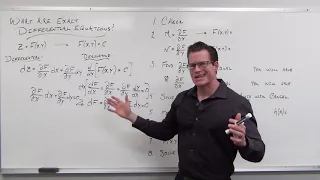What are Exact Differential Equations (Differential Equations 28)

https://www.patreon.com/ProfessorLeonard An explanation of the origin, use, and solving of Exact Differential Equations

From playlist Differential Equations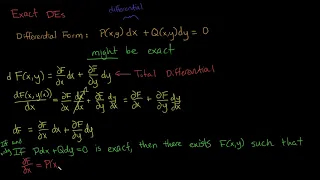Differential Equations: Exact DEs Introduction 1

The third analytic method for solving first order differential equations only works on exact differential equations. In this video, part one of the introduction, I explain what it means for a differential equation to be exact and why it's useful for solving it.

From playlist Differential Equations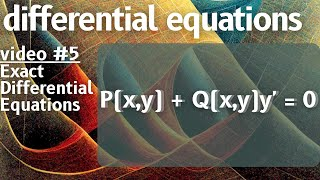Exact Equations -- Differential Equations 5

⭐Support the channel⭐ Patreon: https://www.patreon.com/michaelpennmath Merch: https://teespring.com/stores/michael-penn-math My amazon shop: https://www.amazon.com/shop/michaelpenn 🟢 Discord: https://discord.gg/Ta6PTGtKBm ⭐my other channels⭐ Main Channel: https://www.youtube.

From playlist Differential Equations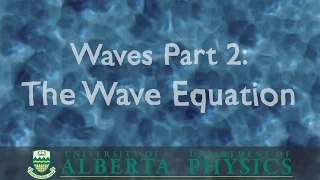PHYS 146 Waves part 2: The Wave Equation

Video lecture for PHYS 146 at the University of Alberta. Shows the derivation of the wave equation from first principles.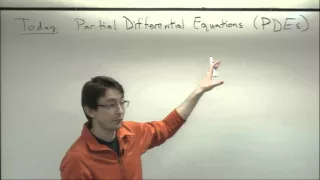ME565 Lecture 7: Canonical Linear PDEs: Wave equation, Heat equation, and Laplace's equation

ME565 Lecture 7 Engineering Mathematics at the University of Washington Canonical Linear PDEs: Wave equation, Heat equation, and Laplace's equation Notes: http://faculty.washington.edu/sbrunton/me565/pdf/L07.pdf Course Website: http://faculty.washington.edu/sbrunton/me565/ http://fac

From playlist Engineering Mathematics (UW ME564 and ME565)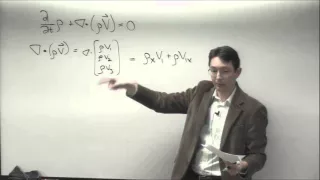ME564 Lecture 24: Directional derivative, continuity equation, and examples of vector fields

ME564 Lecture 24 Engineering Mathematics at the University of Washington Directional derivative, continuity equation, and examples of vector fields Notes: http://faculty.washington.edu/sbrunton/me564/pdf/L24.pdf Course Website: http://faculty.washington.edu/sbrunton/me564/ http://facult

From playlist Engineering Mathematics (UW ME564 and ME565)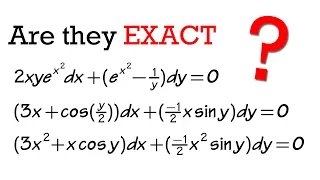EXACT Equations (how to check for exactness!) 3 examples

How to check if a differential equation is exact or not? How to check for exactness? Solve exact equations, Part1 of Differential Equation Course: How to solve first order differential equations? The topics/technique include: separable differential equations, first order linear diffe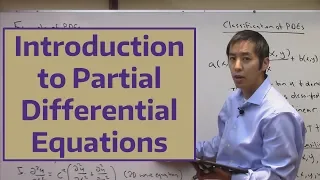Introduction to Partial Differential Equations

This is the first lesson in a multi-video discussion focused on partial differential equations (PDEs). In this video we introduce PDEs and compare them with ordinary differential equations (ODEs). We investigate how PDEs introduce additional complexity and richness to an engineering appl

From playlist Partial Differential Equations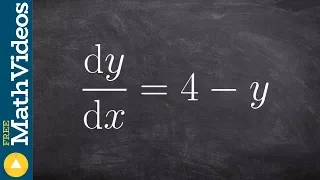Solve differentiable equations with In

Learn how to solve the particular solution of differential equations. A differential equation is an equation that relates a function with its derivatives. The solution to a differential equation involves two parts: the general solution and the particular solution. The general solution give

From playlist Differential Equations